This book is archived and will be removed July 6, 2022. Please use the updated version.

Quantum Mechanics

# 53 The Quantum Particle in a Box

### Learning Objectives

By the end of this section, you will be able to:

• Describe how to set up a boundary-value problem for the stationary Schrӧdinger equation
• Explain why the energy of a quantum particle in a box is quantized
• Describe the physical meaning of stationary solutions to Schrӧdinger’s equation and the connection of these solutions with time-dependent quantum states
• Explain the physical meaning of Bohr’s correspondence principle

In this section, we apply Schrӧdinger’s equation to a particle bound to a one-dimensional box. This special case provides lessons for understanding quantum mechanics in more complex systems. The energy of the particle is quantized as a consequence of a standing wave condition inside the box.

Consider a particle of mass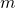that is allowed to move only along the x-direction and its motion is confined to the region between hard and rigid walls located at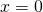and at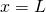((Figure)). Between the walls, the particle moves freely. This physical situation is called the infinite square well, described by the potential energy function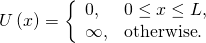Combining this equation with Schrӧdinger’s time-independent wave equation gives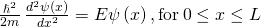where E is the total energy of the particle. What types of solutions do we expect? The energy of the particle is a positive number, so if the value of the wave function is positive (right side of the equation), the curvature of the wave function is negative, or concave down (left side of the equation). Similarly, if the value of the wave function is negative (right side of the equation), the curvature of the wave function is positive or concave up (left side of equation). This condition is met by an oscillating wave function, such as a sine or cosine wave. Since these waves are confined to the box, we envision standing waves with fixed endpoints atand.

The potential energy function that confines the particle in a one-dimensional box.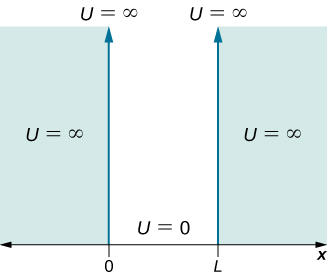Solutions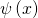to this equation have a probabilistic interpretation. In particular, the square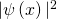represents the probability density of finding the particle at a particular location x. This function must be integrated to determine the probability of finding the particle in some interval of space. We are therefore looking for a normalizable solution that satisfies the following normalization condition: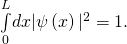The walls are rigid and impenetrable, which means that the particle is never found beyond the wall. Mathematically, this means that the solution must vanish at the walls: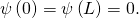We expect oscillating solutions, so the most general solution to this equation is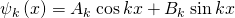where k is the wave number, and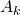and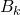are constants. Applying the boundary condition expressed by (Figure) gives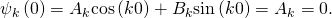Because we have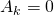, the solution must be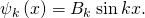Ifis zero,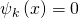for all values of x and the normalization condition, (Figure), cannot be satisfied. Assuming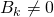, (Figure) forthen gives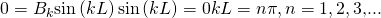We discard the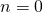solution becausefor this quantum number would be zero everywhere—an un-normalizable and therefore unphysical solution. Substituting (Figure) into (Figure) gives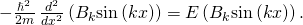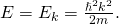According to de Broglie,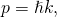so this expression implies that the total energy is equal to the kinetic energy, consistent with our assumption that the “particle moves freely.” Combining the results of (Figure) and (Figure) gives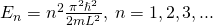Strange! A particle bound to a one-dimensional box can only have certain discrete (quantized) values of energy. Further, the particle cannot have a zero kinetic energy—it is impossible for a particle bound to a box to be “at rest.”

To evaluate the allowed wave functions that correspond to these energies, we must find the normalization constant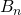. We impose the normalization condition (Figure) on the wave function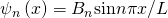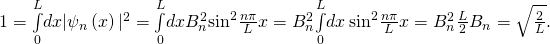Hence, the wave functions that correspond to the energy values given in (Figure) are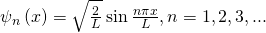For the lowest energy state or ground state energy, we have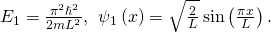All other energy states can be expressed as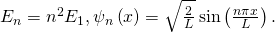The index n is called the energy quantum number or principal quantum number. The state for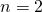is the first excited state, the state for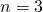is the second excited state, and so on. The first three quantum states (for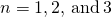of a particle in a box are shown in (Figure).

The wave functions in (Figure) are sometimes referred to as the “states of definite energy.” Particles in these states are said to occupy energy levels, which are represented by the horizontal lines in (Figure). Energy levels are analogous to rungs of a ladder that the particle can “climb” as it gains or loses energy.

The wave functions in (Figure) are also called stationary states and standing wave states. These functions are “stationary,” because their probability density functions,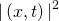, do not vary in time, and “standing waves” because their real and imaginary parts oscillate up and down like a standing wave—like a rope waving between two children on a playground. Stationary states are states of definite energy [(Figure)], but linear combinations of these states, such as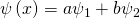(also solutions to Schrӧdinger’s equation) are states of mixed energy.

The first three quantum states of a quantum particle in a box for principal quantum numbers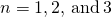: (a) standing wave solutions and (b) allowed energy states.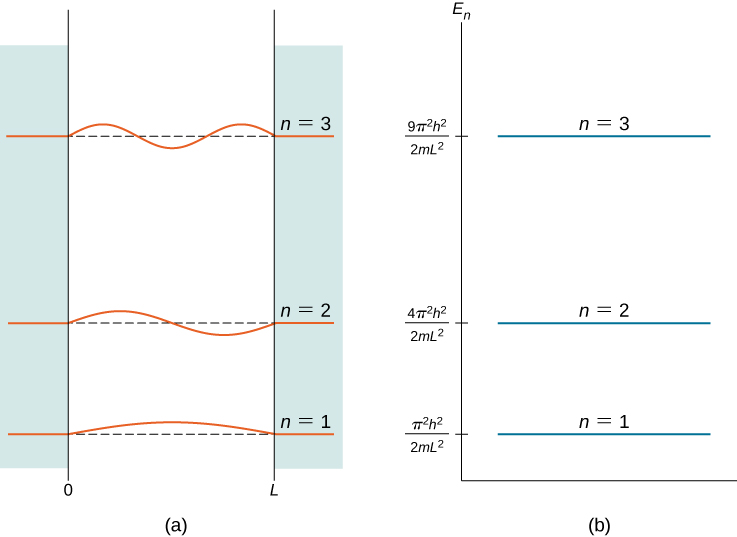Energy quantization is a consequence of the boundary conditions. If the particle is not confined to a box but wanders freely, the allowed energies are continuous. However, in this case, only certain energies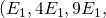…) are allowed. The energy difference between adjacent energy levels is given by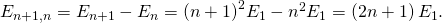Conservation of energy demands that if the energy of the system changes, the energy difference is carried in some other form of energy. For the special case of a charged particle confined to a small volume (for example, in an atom), energy changes are often carried away by photons. The frequencies of the emitted photons give us information about the energy differences (spacings) of the system and the volume of containment—the size of the “box” [see (Figure)].

A Simple Model of the Nucleus Suppose a proton is confined to a box of width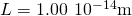(a typical nuclear radius). What are the energies of the ground and the first excited states? If the proton makes a transition from the first excited state to the ground state, what are the energy and the frequency of the emitted photon?

Strategy If we assume that the proton confined in the nucleus can be modeled as a quantum particle in a box, all we need to do is to use (Figure) to find its energies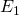and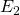. The mass of a proton is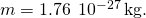The emitted photon carries away the energy difference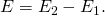We can use the relation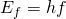to find its frequency f.

Solution The ground state: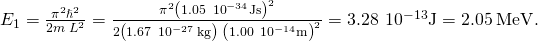The first excited state: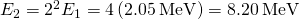.

The energy of the emitted photon is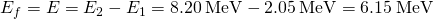.

The frequency of the emitted photon is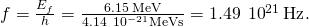Significance This is the typical frequency of a gamma ray emitted by a nucleus. The energy of this photon is about 10 million times greater than that of a visible light photon.

The expectation value of the position for a particle in a box is given by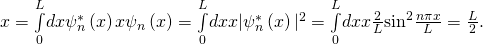We can also find the expectation value of the momentum or average momentum of a large number of particles in a given state: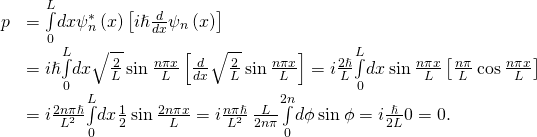Thus, for a particle in a state of definite energy, the average position is in the middle of the box and the average momentum of the particle is zero—as it would also be for a classical particle. Note that while the minimum energy of a classical particle can be zero (the particle can be at rest in the middle of the box), the minimum energy of a quantum particle is nonzero and given by (Figure). The average particle energy in the nth quantum state—its expectation value of energy—is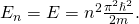The result is not surprising because the standing wave state is a state of definite energy. Any energy measurement of this system must return a value equal to one of these allowed energies.

Our analysis of the quantum particle in a box would not be complete without discussing Bohr’s correspondence principle. This principle states that for large quantum numbers, the laws of quantum physics must give identical results as the laws of classical physics. To illustrate how this principle works for a quantum particle in a box, we plot the probability density distribution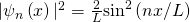for finding the particle around location x between the walls when the particle is in quantum state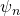. (Figure) shows these probability distributions for the ground state, for the first excited state, and for a highly excited state that corresponds to a large quantum number. We see from these plots that when a quantum particle is in the ground state, it is most likely to be found around the middle of the box, where the probability distribution has the largest value. This is not so when the particle is in the first excited state because now the probability distribution has the zero value in the middle of the box, so there is no chance of finding the particle there. When a quantum particle is in the first excited state, the probability distribution has two maxima, and the best chance of finding the particle is at positions close to the locations of these maxima. This quantum picture is unlike the classical picture.

The probability density distribution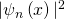for a quantum particle in a box for: (a) the ground state,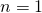; (b) the first excited state,; and, (c) the nineteenth excited state,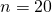.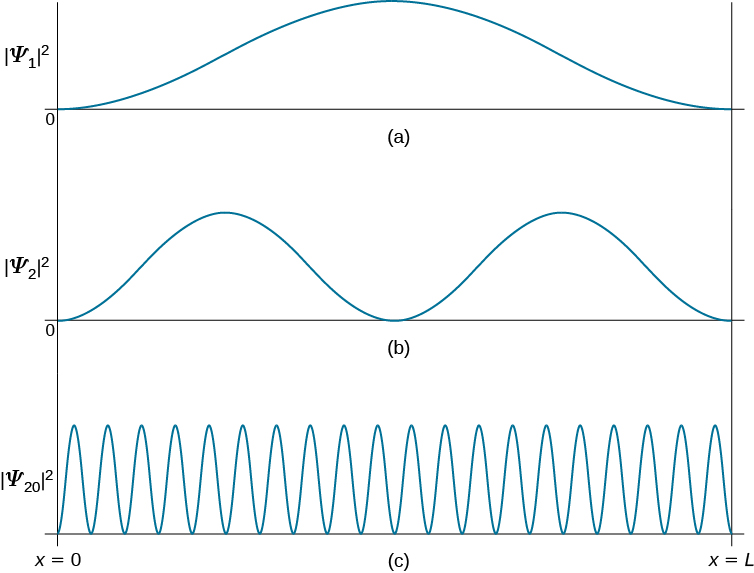The probability density of finding a classical particle between x and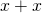depends on how much timethe particle spends in this region. Assuming that its speed u is constant, this time is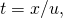which is also constant for any location between the walls. Therefore, the probability density of finding the classical particle at x is uniform throughout the box, and there is no preferable location for finding a classical particle. This classical picture is matched in the limit of large quantum numbers. For example, when a quantum particle is in a highly excited state, shown in (Figure), the probability density is characterized by rapid fluctuations and then the probability of finding the quantum particle in the interval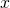does not depend on where this interval is located between the walls.

A Classical Particle in a Box A small 0.40-kg cart is moving back and forth along an air track between two bumpers located 2.0 m apart. We assume no friction; collisions with the bumpers are perfectly elastic so that between the bumpers, the car maintains a constant speed of 0.50 m/s. Treating the cart as a quantum particle, estimate the value of the principal quantum number that corresponds to its classical energy.

Strategy We find the kinetic energy K of the cart and its ground state energyas though it were a quantum particle. The energy of the cart is completely kinetic, so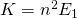((Figure)). Solving for n gives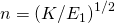.

Solution The kinetic energy of the cart is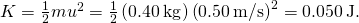The ground state of the cart, treated as a quantum particle, is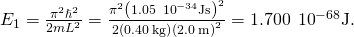Therefore,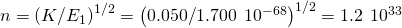.

Significance We see from this example that the energy of a classical system is characterized by a very large quantum number. Bohr’s correspondence principle concerns this kind of situation. We can apply the formalism of quantum mechanics to any kind of system, quantum or classical, and the results are correct in each case. In the limit of high quantum numbers, there is no advantage in using quantum formalism because we can obtain the same results with the less complicated formalism of classical mechanics. However, we cannot apply classical formalism to a quantum system in a low-number energy state.

Check Your Understanding (a) Consider an infinite square well with wall boundariesand. What is the probability of finding a quantum particle in its ground state somewhere betweenand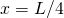? (b) Repeat question (a) for a classical particle.

a. 9.1%; b. 25%

Having found the stationary states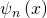and the energies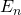by solving the time-independent Schrӧdinger equation (Figure), we use (Figure) to write wave functions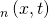that are solutions of the time-dependent Schrӧdinger’s equation given by (Figure). For a particle in a box this gives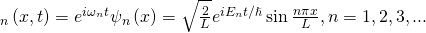where the energies are given by (Figure).

The quantum particle in a box model has practical applications in a relatively newly emerged field of optoelectronics, which deals with devices that convert electrical signals into optical signals. This model also deals with nanoscale physical phenomena, such as a nanoparticle trapped in a low electric potential bounded by high-potential barriers.

### Summary

• Energy states of a quantum particle in a box are found by solving the time-independent Schrӧdinger equation.
• To solve the time-independent Schrӧdinger equation for a particle in a box and find the stationary states and allowed energies, we require that the wave function terminate at the box wall.
• Energy states of a particle in a box are quantized and indexed by principal quantum number.
• The quantum picture differs significantly from the classical picture when a particle is in a low-energy state of a low quantum number.
• In the limit of high quantum numbers, when the quantum particle is in a highly excited state, the quantum description of a particle in a box coincides with the classical description, in the spirit of Bohr’s correspondence principle.

### Conceptual Questions

Using the quantum particle in a box model, describe how the possible energies of the particle are related to the size of the box.

Is it possible that when we measure the energy of a quantum particle in a box, the measurement may return a smaller value than the ground state energy? What is the highest value of the energy that we can measure for this particle?

No. For an infinite square well, the spacing between energy levels increases with the quantum number n. The smallest energy measured corresponds to the transition from n = 2 to 1, which is three times the ground state energy. The largest energy measured corresponds to a transition from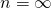to 1, which is infinity. (Note: Even particles with extremely large energies remain bound to an infinite square well—they can never “escape”)

For a quantum particle in a box, the first excited state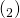has zero value at the midpoint position in the box, so that the probability density of finding a particle at this point is exactly zero. Explain what is wrong with the following reasoning: “If the probability of finding a quantum particle at the midpoint is zero, the particle is never at this point, right? How does it come then that the particle can cross this point on its way from the left side to the right side of the box?

### Problems

Assume that an electron in an atom can be treated as if it were confined to a box of width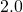. What is the ground state energy of the electron? Compare your result to the ground state kinetic energy of the hydrogen atom in the Bohr’s model of the hydrogen atom.

9.4 eV, 64%

Assume that a proton in a nucleus can be treated as if it were confined to a one-dimensional box of width 10.0 fm. (a) What are the energies of the proton when it is in the states corresponding to,, and? (b) What are the energies of the photons emitted when the proton makes the transitions from the first and second excited states to the ground state?

An electron confined to a box has the ground state energy of 2.5 eV. What is the width of the box?

0.38 nm

What is the ground state energy (in eV) of a proton confined to a one-dimensional box the size of the uranium nucleus that has a radius of approximately 15.0 fm?

What is the ground state energy (in eV) of an-particle confined to a one-dimensional box the size of the uranium nucleus that has a radius of approximately 15.0 fm?

1.82 MeV

To excite an electron in a one-dimensional box from its first excited state to its third excited state requires 20.0 eV. What is the width of the box?

An electron confined to a box of width 0.15 nm by infinite potential energy barriers emits a photon when it makes a transition from the first excited state to the ground state. Find the wavelength of the emitted photon.

24.7 nm

If the energy of the first excited state of the electron in the box is 25.0 eV, what is the width of the box?

Suppose an electron confined to a box emits photons. The longest wavelength that is registered is 500.0 nm. What is the width of the box?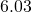Hydrogen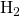molecules are kept at 300.0 K in a cubical container with a side length of 20.0 cm. Assume that you can treat the molecules as though they were moving in a one-dimensional box. (a) Find the ground state energy of the hydrogen molecule in the container. (b) Assume that the molecule has a thermal energy given by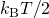and find the corresponding quantum number n of the quantum state that would correspond to this thermal energy.

An electron is confined to a box of width 0.25 nm. (a) Draw an energy-level diagram representing the first five states of the electron. (b) Calculate the wavelengths of the emitted photons when the electron makes transitions between the fourth and the second excited states, between the second excited state and the ground state, and between the third and the second excited states.

a.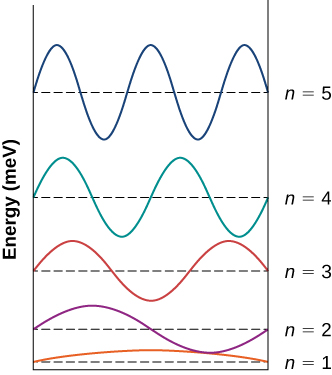;
b.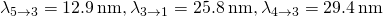An electron in a box is in the ground state with energy 2.0 eV. (a) Find the width of the box. (b) How much energy is needed to excite the electron to its first excited state? (c) If the electron makes a transition from an excited state to the ground state with the simultaneous emission of 30.0-eV photon, find the quantum number of the excited state?

### Glossary

energy levels
states of definite energy, often represented by horizontal lines in an energy “ladder” diagram
energy quantum number
index that labels the allowed energy states
ground state energy
lowest energy state in the energy spectrum
infinite square well
potential function that is zero in a fixed range and infinitely beyond this range
principal quantum number
energy quantum number
standing wave state
stationary state for which the real and imaginary parts of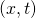oscillate up and down like a standing wave (often modeled with sine and cosine functions)
stationary state
state for which the probability density function,, does not vary in time